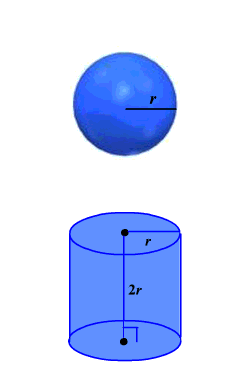# Surface Area of a Sphere

The Greek mathematician Archimedes discovered that the surface area of a sphere is the same as the lateral surface area of a cylinder having the same radius as the sphere and a height the length of the diameter of the sphere.The lateral surface area of the cylinder is $2\pi rh$ where $h=2r$ .

Lateral Surface Area of the Cylinder $=2\pi r\left(2r\right)=4\pi {r}^{2}$ .

Therefore, the Surface Area of a Sphere with radius $r$ equals $4\pi {r}^{2}$ .

Example :

Find the surface area of a sphere with radius $5$ inches.

$S.A.=4\pi {\left(5\right)}^{2}=100\pi \text{\hspace{0.17em}}{\text{inches}}^{2}\approx 314.16\text{\hspace{0.17em}}{\text{inches}}^{2}$